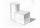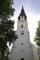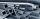# Solid geometry, stereometry

Solid geometry is the name for the geometry of three-dimensional Euclidean space.

Stereometry deals with the measurements of volumes of various solid figures (three-dimensional figures) including pyramids, prisms and other polyhedrons; cylinders; cones; truncated cones; and balls bounded by spheres.

1. Tetrahedral prism - rhomboid baseCalculate the area and volume tetrahedral prism that has base rhomboid shape and its dimensions are: a = 12 cm, b = 70 mm, v_a = 6 cm, v_h = 1 dm.Find the volume and surface of a regular quadrilateral pyramid if the bottom edge is 45 cm long and the pyramid height is 7 cm.
3. Hexagon rotationA regular hexagon of side 6 cm is rotated through 60° along a line passing through its longest diagonal. What is the volume of the figure thus generated?
4. Pool in litresPool has a width of 3.5 m length of 6 m and a height 1.60 meters. Calculate pool volume in liters.
5. Painting a hutIt is necessary to paint the exterior walls of hut whose layout is a rectangle of 6.16 m x 8.78 m wall height is 2.85 meters. Cottage has five rectangular windows; three have dimensions of 1.15 m x 1.32 m and two 0,45 m x 0.96 m. How many m2 is necessar
6. Surface area of cylinderDetermine the lateral surface of the rotary cylinder which is circumscribed cube with edge length 5 cm.
7. VintnerHow high can vintner fill keg with crushed red grapes if these grapes occupy a volume of 20 percent? Keg is cylindrical with a diameter of the base 1 m and a volume 9.42 hl. Start from the premise that says that fermentation will fill the keg (the number
8. Surface and volume od cuboidContent area of the square base of cuboid is Sp = 36 cm2 and its height 80 mm. Determine its surface area and volume.
9. Tetrahedral pyramidIt is given a regular tetrahedral pyramid with base edge 6 cm and the height of the pyramid 10 cm. Calculate the length of its side edges.
10. Horizontal Cylindrical SegmentHow much fuel is in the tank of horizontal cylindrical segment with a length 10m, width of level 1 meter and level is 0.2 meters below the upper side of the tank?
11. Triangular prismThe perpendicular triangular prism is a right triangle with a 5 cm leg. The content of the largest wall of the prism is 130 cm2 and the body height is 10 cm. Calculate the body volume.
12. ConeCalculate the volume of the rotating cone with a base radius 26.3 cm and a side 38.4 cm long.
13. Cuboid - edgesThe cuboid has dimensions in ratio 4: 3: 5, the shortest edge is 12 cm long. Find: (A) the lengths of the remaining edges, (B) the surface of the cuboid, (C) the volume of the cuboid
14. Church roofThe roof of the church tower has the shape of a regular tetrahedral pyramid with base edge length 5.4 meters and a height 5 m. It was found that needs to be corrected 27% covering of the roof area. What amount of material will be required?
15. Hexagonal prism 2The regular hexagonal prism has a surface of 140 cm2 and height of 5 cm. Calculate its volume.
16. The factoryThe factory ordered 500 hexagonal steel bars of square section with 25 mm side. How many cars with a load capacity of 3 tonnes will be needed for bars move if the steel density is 7,850 kg.m-3?
17. Cube wallsFind the volume and surface area of the cube if the area of one wall is 40cm2.The regular quadrangular prism has a base edge a = 7.1 cm and side edge = 18.2 cm long. Calculate its volume and surface area.
19. Pyramid in cubeIn a cube with edge 12 dm long we have inscribed pyramid with the apex at the center of the upper wall of the cube. Calculate the volume and surface area of the pyramid.
20. Sphere vs cubeHow many % of the surface of a sphere of radius 12 cm is the surface of a cube inscribed in this sphere?

Do you have an interesting mathematical word problem that you can't solve it? Submit math problem, and we can try to solve it.

We will send a solution to your e-mail address. Solved examples are also published here. Please enter the e-mail correctly and check whether you don't have a full mailbox.

Please do not submit problems from current active competitions such as Mathematical Olympiad, correspondence seminars etc...